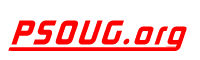Quick Search: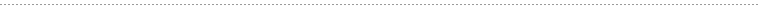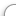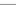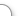CODE Oracle PL/SQL Code Library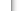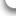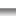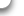JOBS Find Or Post Oracle JobsFORUM Oracle Discussion & ChatPHP Code: Convert miles to feet, feet to miles, or inches. Jump to: Select a Location PSOUG Home Page Oracle Code Library mySQL Code Library PHP Code Library JavaScript Code Library Oracle Terms & Definitions Oracle Error Codes PSOUG Community Blogs Oracle Jobs Board PSOUG Forum Oracle User Group Directory Free Oracle Magazines Online Learning Center PSOUG Presentations Advanced Code Search News and Events Sponsors Page Submit Code Contact UsSnippet Name: Convert miles to feet, feet to miles, or inches.

Description: Handy function to convert miles to feet, feet to miles, miles to inches, etc.

Comment: (none)

Language: PHP
Highlight Mode: PHP

```
<?PHP
// position 1 = distance numeric numbers only no commas
// position 2 = current length type of position 1
// position 3 = convert length type of position 1 and 2
// position 4 = FT,MI,IN extentions on or off :: 1=on : 2=off
// m = miles
// f = feet
// i = inches

FUNCTION distance(\$curlen,\$type,\$totype,\$on){

// Check to see if the first value is an integer
IF(!IS_INT(\$curlen)){
RETURN 'Wrong input type for first value';
EXIT;
}

// Make sure second value is either f,i, or m
IF(\$type!="f"&&\$type!="i"&&\$type!="m"){
RETURN 'Wrong input type for second value';
EXIT;
}

// Make sure third value is either f,i, or m
IF(\$totype!="f"&&\$totype!="i"&&\$totype!="m"){
RETURN 'Wrong input type for third value';
EXIT;
}

// Make sure fourth value is either 1 or 2
IF(\$on!=1&&\$on!=2){
RETURN 'Wrong input type for fourth value';
EXIT;
}

// If it validate the tests above, get the current type
SWITCH(\$type){
// if it is an i do the math
CASE "i":
IF(\$totype=="f"){
\$len = \$curlen / 12;
IF(\$on==1){
RETURN NUMBER_FORMAT(ROUND(\$len,1)).' FT';
}ELSE{
RETURN NUMBER_FORMAT(ROUND(\$len,1));
}
}ELSEIF(\$totype=="m"){
\$len = \$curlen / 63360;
IF(\$on==1){
RETURN NUMBER_FORMAT(ROUND(\$len,1)).' MI';
}ELSE{
RETURN NUMBER_FORMAT(ROUND(\$len,1));
}
}
BREAK;
// if it is an f do the math
CASE "f":
IF(\$totype=="i"){
\$len = \$curlen * 12;
IF(\$on==1){
RETURN NUMBER_FORMAT(ROUND(\$len,1)).' IN';
}ELSE{
RETURN NUMBER_FORMAT(ROUND(\$len,1));
}
}ELSEIF(\$totype=="m"){
\$len = \$curlen / 5280;
IF(\$on==1){
RETURN NUMBER_FORMAT(ROUND(\$len,1)).' MI';
}ELSE{
RETURN NUMBER_FORMAT(ROUND(\$len,1));
}
}
BREAK;
// if it is an m do the math
CASE "m":
IF(\$totype=="i"){
\$len = \$curlen * 63360;
IF(\$on==1){
RETURN NUMBER_FORMAT(ROUND(\$len,1)).' IN';
}ELSE{
RETURN NUMBER_FORMAT(ROUND(\$len,1));
}
}ELSEIF(\$totype=="f"){
\$len = \$curlen * 5280;
IF(\$on==1){
RETURN NUMBER_FORMAT(ROUND(\$len,1)).' FT';
}ELSE{
RETURN NUMBER_FORMAT(ROUND(\$len,1));
}
}
BREAK;
}
}

ECHO distance(123,"m","f",1);

?> ```FreeOracle MagazineSubscriptionsand Oracle White Papers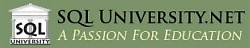SQL University.net courses meet the most demanding needs of the business world for advanced education in a cost-effective manner. SQL University.net courses are available immediately for IT professionals and can be taken without disruption of your workplace schedule or processes.

Compared to traditional travel-based training, SQL University.net saves time and valuable corporate resources, allowing companies to do more with less. That's our mission, and that's what we deliver.